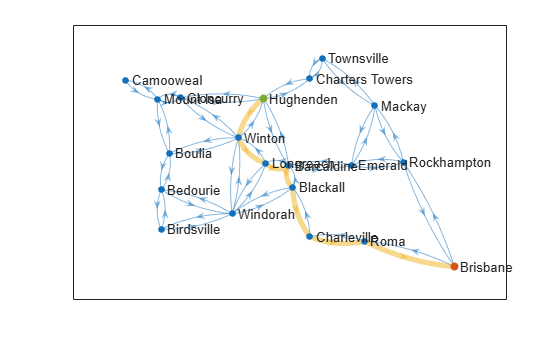# plannerAStar

Graph-based A* path planner

Since R2023a

## Description

The `plannerAStar` object creates an A* path planner from a graph object. The A* algorithm finds the shortest path in the graph by using a heuristic function to efficiently guide its exploration of the nodes.

## Creation

### Syntax

``planner = plannerAStar(graph)``
``planner = plannerAStar(___,Name=Value)``

### Description

example

````planner = plannerAStar(graph)` creates a `plannerAStar` object from a `navGraph` object, `graph`. The `graph` input sets the value of the Graph property.```
````planner = plannerAStar(___,Name=Value)` sets properties using one or more name-value arguments in addition to the input argument in the previous syntax. You can specify the HeuristicCostFcn and TieBreaker properties as name-value arguments.```

## Properties

expand all

Graph object of the planning environment, specified as a `navGraph` object. If using a `digraph` object, you must first convert it to a `navGraph` object. The planner uses the link (or edge) weights from the `navGraph` object to compute the path cost.

The heuristic cost between a state and the goal in a graph, specified as one of the predefined cost function handles, `@nav.algs.distanceManhattan`, `@nav.algs.distanceEuclidean`, or `@nav.algs.distanceEuclideanSquared`, or a custom cost function handle.

The cost function must accept two N-by-S matrices, `state1` and `state2`, where N is the number of states, and S is the length of the state vector. The function must return an N-element column vector of heuristic costs, `H`. If N is the same for both inputs, then `H` contains the pairwise costs between the states. Otherwise, one matrix must have a single row, and `H` contains the cost between that state and each state in the opposing matrix. Vectorize the custom cost function for best performance.

Example: `HeuristicCostFcn=@nav.algs.distanceEuclidean`

Example: `HeuristicCostFcn=@(state1,state2)nav.algs.distanceManhattan(state1,state2,weight)`

Example: `HeuristicCostFcn=@(state1,state2)sqrt(sum((state1-state2).^2,2))`

Data Types: `function_handle`

Tiebreaker mode toggle, specified as either a logical `0` (`false`) or `1` (`true`). When you enable the `TieBreaker` property, the A* path planner chooses between multiple paths of the same length by adjusting the heuristic cost value.

Example: `TieBreaker=true`

Data Types: `logical`

## Object Functions

 `plan` Find shortest path between two states in graph `copy` Create deep copy of A* path planner object

## Examples

collapse all

`load("queenslandRoutes","places","routes")`

Specify states, links, and weights for a `navGraph` object.

```states = places.utm; % UTM coordinates of cities names = places.name; % Names of cities links = [routes.start routes.end]; % Adjacent cities weights = routes.time; % Travel time between adjacent cities```

Create a `navGraph` object.

```graphObj = navGraph(states,links,Weight=weights, ... Name=names);```

Create a graph-based A* path planner.

`planner = plannerAStar(graphObj);`

Create a deep copy of the `plannerAStar` object.

`planner2 = copy(planner)`
```planner2 = plannerAStar with properties: HeuristicCostFcn: @nav.algs.distanceManhattan TieBreaker: 0 Graph: [1x1 navGraph] ```

Specify a heuristic function returns an estimated time to reach the goal.

```planner.HeuristicCostFcn = @(state1,state2) ... sum(abs(state1-state2),2)/100;```

Define the start and goal cities.

```start = "Hughenden"; goal = "Brisbane";```

Find the shortest path using the graph-based A* algorithm.

`[pathOutput,solutionInfo] = plan(planner,start,goal);`

Visualize the results.

```h = show(graphObj); set(h,XData=graphObj.States.StateVector(:,1), ... YData=graphObj.States.StateVector(:,2)) pathStateIDs = solutionInfo.PathStateIDs; highlight(h,pathStateIDs,EdgeColor="#EDB120",LineWidth=4) highlight(h,pathStateIDs(1),NodeColor="#77AC30",MarkerSize=5) highlight(h,pathStateIDs(end),NodeColor="#D95319",MarkerSize=5)```## Version History

Introduced in R2023a